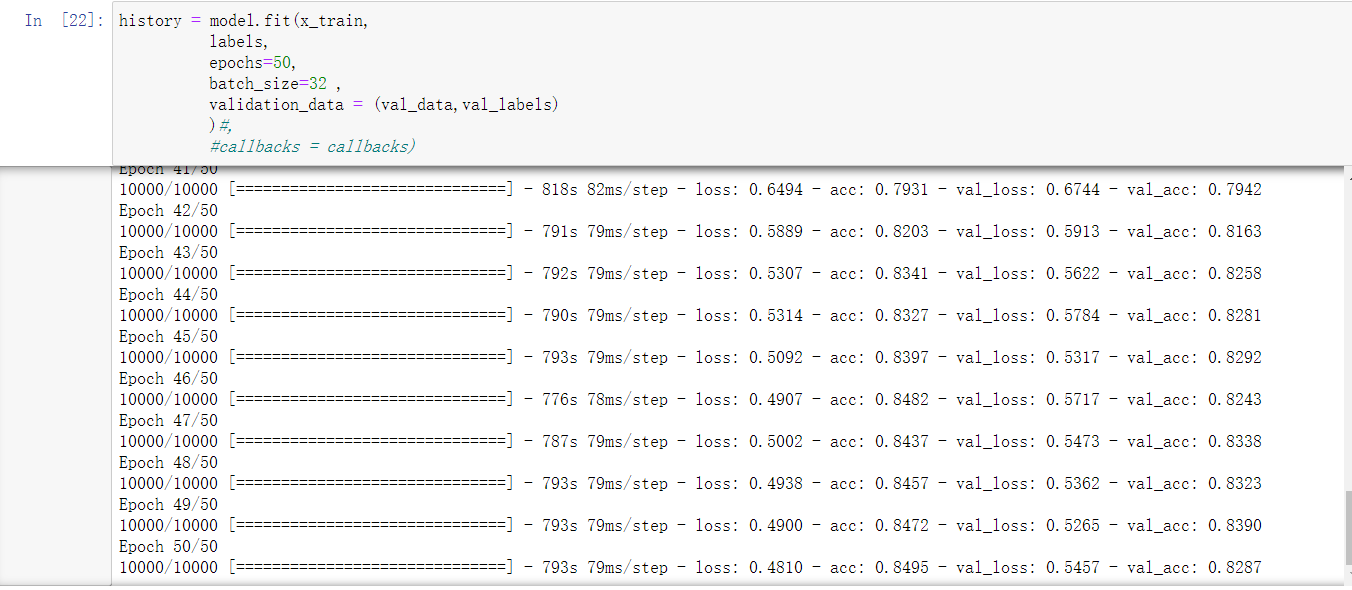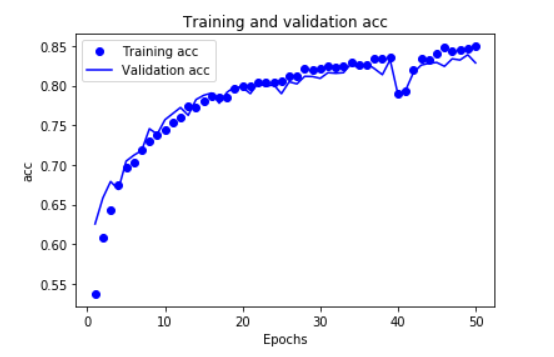# 简单使用深度学习进行恶意代码检测

alert1   ·   发表于 2021-01-31 10:51:33   ·   技术文章## 数据加载和转储

### 测试集数据

``数据全部读取``#读取训练集文件``labels = [] #标签``files = []  #训练集api调用序列``print('读取训练集')``data = pd.read_csv(train_path)#,nrows=15000000) #将训练集的前nrows行读取并保存到data中``goup_fileid = data.groupby('file_id')         #将data按照file_id进行分组``for file_name, file_group in goup_fileid:     #将标签和调用顺序提取出来分别保存到labels和files两个列表中``    #print(file_name)``    file_labels = file_group['label'].values``    result = file_group.sort_values(['tid', 'index'], ascending=True)``    api_sequence = ' '.join(result['api'])``    labels.append(str(file_labels))``    files.append(api_sequence)``#print(result)``#print(labels)``#print(files)``with open("security_train_labels.json", 'w') as f:  #分别将labels和files序列化保存在两个不同的json中``    json.dump(labels, f)``with open("security_train_files.json", 'w') as f:``    json.dump(files, f)``print('读取完毕')``

## 加载json文件中的数据

``with open("security_train_labels.json", "r") as f:    #加载处理完的json文件``    labels = json.load(f)                              #标签``with open("security_train_files.json", "r") as f:``    files = json.load(f)                               #训练集api调用序列``#with open("security_test_names.json", "r") as f:``#    file_names = json.load(f)                          #测试集样本序号``with open("security_test_outfiles.json", "r") as f:``    testfiles = json.load(f)                           #测试集api调用序列``

## 数据处理

### api调用长序列处理

``labels = np.asarray(labels)``labels = to_categorical(labels)``tokenizer = Tokenizer()    #为测试集和训练集所有api产生一个索引``tokenizer.fit_on_texts(files)``tokenizer.fit_on_texts(testfiles)``maxlen = 6000             #单个恶意代码的api序列长度最大为6000``
``index = tokenizer.word_index``x_train_word = tokenizer.texts_to_sequences(files)   #将api名转换为tokenizer中的索引``x_out_word = tokenizer.texts_to_sequences(testfiles)``x_train = preprocessing.sequence.pad_sequences(x_train_word, maxlen=maxlen)#将整数列表转换为整数张量``x_test = preprocessing.sequence.pad_sequences(x_out_word, maxlen=maxlen)``val_data = x_train[10000:]``x_train = x_train[:10000]``val_labels = labels[10000:]``labels= labels[:10000]``

## 训练模型

### 层的堆叠

LSTM层（长短期记忆）减轻了RNN中的梯度消失问题，是比较好的处理有顺序的模型，因为它可以获取前面的信息，同时还有GRU层，他是rnn和lstm的折中

### 词嵌入

(180,6000) -> (180,6000,256)
eg.比如单词：baby 可能经过训练后可由[-0.2,0.1,0.15,0.24,0.45]这样一个向量表示，虽然每个位置单独没有意义，但是这样构成的向量的确是可以表示这一个单词的。

### 序列数据的一维池化

``model = Sequential()``model.add(layers.Embedding(304,256,input_length=maxlen,name = 'embed'))``model.add(layers.Conv1D(64,9,padding='same',activation='relu'))``model.add(layers.Conv1D(64,9,padding='same',activation='relu'))``model.add(layers.Conv1D(64,9,padding='same',activation='relu'))``model.add(layers.MaxPooling1D(3))``model.add(layers.Conv1D(64,9,padding='same',activation='relu'))``model.add(layers.Conv1D(64,9,padding='same',activation='relu'))``model.add(layers.Conv1D(64,9,padding='same',activation='relu'))``model.add(layers.MaxPooling1D())``model.add(layers.LSTM(64,dropout=0.1,recurrent_dropout=0.5,return_sequences=True))``model.add(layers.LSTM(64,dropout=0.1,recurrent_dropout=0.5))``#model.add(layers.Bidirectional(layers.LSTM(64,dropout=0.1,recurrent_dropout=0.5)))``#model.add(layers.Dense(32,activation = 'relu'))#,input_shape = (4,)))``model.add(layers.Dense(8,activation = 'softmax'))``### 优化器

rmsprop优化器通常都是足够好的选择，同时也使用了它默认的学习率

### 训练指标

accuracy精度，衡量成功的指标

### 训练轮数和训练批次

``model.compile(optimizer=RMSprop(lr=1e-4),loss='categorical_crossentropy',metrics=['acc']) #adma优化器 losslog  lr=1e-4``history = model.fit(x_train,``          labels,``          epochs=50,``          batch_size=32 ,``          validation_data = (val_data,val_labels)``          )#,``          #callbacks = callbacks)``

histogram_freq参数会在面板中添加histograms面板和distributions面板，会将每一个层的权值、偏置、输出值的统计分布以不同的视觉角度显示出来，因为权重等信息的存在，现在日志文件变为了111M，增大了很多。

``#可视化``callbacks = [``    keras.callbacks.TensorBoard(``        log_dir = 'log',``        #histogram_freq=1,#显示histograms和distributions面板``        #histogram_freq = 1,``        #write_graph=True,``        #write_images=True``        #embeddings_freq = 1,``        #embeddings_layer_names = 'embed',``        #embeddings_data=None#.astype(float)``    )``]````history_dict = history.history``acc_values = history_dict['acc']``val_acc_values = history_dict['val_acc']``epochs = range(1,len(acc_values) + 1)``plt.plot(epochs,acc_values,'bo',label = 'Training acc')``plt.plot(epochs,val_acc_values,'b',label = 'Validation acc')``plt.title('Training and validation acc')``plt.xlabel('Epochs')``plt.ylabel('acc')``plt.legend()``plt.show()````history_dict = history.history``loss_values = history_dict['loss']``val_loss_values = history_dict['val_loss']``epochs = range(1,len(loss_values) + 1)``plt.plot(epochs,loss_values,'bo',label = 'Training loss')``plt.plot(epochs,val_loss_values,'b',label = 'Validation loss')``plt.title('Training and validation loss')``plt.xlabel('Epochs')``plt.ylabel('loss')``plt.legend()``plt.show()``### 测试集训练

``model.save('ali1.h5')``#model = tensorflow.keras.models.load_model('ali1.h5')``predictions = model.predict(x_test,batch_size = 1)``test_labels = pd.DataFrame(predictions,``           #index=['a','b','c','d','e']``           index = range(1,len(predictions)+1),``           columns=['prob0','prob1','prob2','prob3','prob4','prob5','prob6','prob7']``         )  # 默认生成整数索引, 字典的键作列,值作行``test_labels.index.name = 'file_id'``test_labels.to_csv('test_labels.csv')``

## 不足与改进

• 数据处理，线程是没有顺序的
• 层数的堆叠不够
• 应该可以使用卷积池化层来处理长序列
• 开发不出来过拟合的模型，难以进行更好的矫正
• 还可以使用一些特征工程上的方法，比如对api的调用数量进行统计，对不同恶意代码的api调用进行分析
• 还可以将数据倒序输入网络进行训练，可能会发现其它特殊的特征
• 调用keras函数式API搭建更复杂有效的网络
• 要使用keras自带的回调函数进行训练，可以进行终止，实时调试等
• 单个模型效果有限，要结合机器学习以及深度学习多模型进行集成

## 绕过方法

可以恶意病毒的调用API过程中随机插入调用一些其他的序列，破坏其API调用的特征和各个API调用序列的整体分布情况，达到绕过的效果。
基于深度学习的方法对序列的长度有着较大的限制，病毒制作者可以一开始先调用一些无关的API，等调用长度超出深度学习检测的限制的时候再开始调用恶意API

veek 120.00 0 2021-01-31 21:09:43 硬核

0 Reply   |  Until 2021-1-31 | 731 View